# Chebyshev system

(diff) ← Older revision | Latest revision (diff) | Newer revision → (diff)
A system of linearly independent functions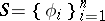in a space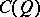with the property that no non-trivial polynomial in this system has more than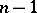distinct zeros. An example of a Chebyshev system in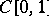is the system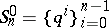,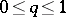; its approximation properties in the uniform metric were first studied by P.L. Chebyshev . The term "Chebyshev system" was introduced by e theory of functions','../c/c025430.htm','Continuation method (to a parametrized family)','../c/c025520.htm','Euler–Lagrange equation','../e/e036510.htm','Fourier series','../f/f041090.htm','Functions of a real variable, theory of','../f/f042130.htm','Hilbert problems','../h/h120080.htm','Jackson inequality','../j/j054000.htm','Laplace theorem','../l/l057530.htm','Lebesgue constants','../l/l057800.htm','Limit theorems','../l/l058920.htm','Linear elliptic partial differential equation and system','../l/l059180.htm','Lyapunov theorem','../l/l061200.htm','Mathematical statistics','../m/m062710.htm','Minimal surface','../m/m063920.htm','Markov–Bernstein-type inequalities','../m/m110060.htm','Ornstein–Uhlenbeck process','../o/o070240.htm','Orthogonal polynomials','../o/o070340.htm','Plateau problem, multi-dimensional','../p/p072850.htm','Quasi-analytic class','../q/q076370.htm')" style="background-color:yellow;">S.N. Bernshtein . An arbitrary Chebyshev system inherits practically all approximation properties of the system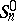.
The Chebyshev theorem and the de la Vallée-Poussin theorem (on alternation) remain valid for Chebyshev systems; all methods developed for the approximate construction of algebraic polynomials of best uniform approximation apply equally well and the uniqueness theorem for polynomials of best uniform approximation is valid for Chebyshev systems (see also Haar condition; Chebyshev set). A compact setadmits a Chebyshev system of degreeif and only if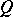is homeomorphic to the circle or to a subset of it (is not homeomorphic to the circle whenis even). In particular, there is no Chebyshev system on any-dimensional domain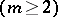, for example on a square .
As an example of a system that is not a Chebyshev system, one can mention the system consisting of splines (cf. Spline) of degreewithfixed knots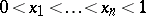. In this case the function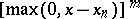belongs to the system, and has infinitely many zeros. Lack of uniqueness makes the numerical construction of best approximations difficult.